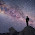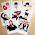# Class VII NCERT (CBSE) - Mathematics

### CBSE Guide NCERT Solutions - Class 7 Mathematics

You have landed at the correct page if you are searching for Class 7 Cbse Ncert Solution of Mathematics | 7th NCERT Math answers | NCERT Solutions and Guide for Class VII Mathematics.

The Table is given after below the Ad -

Class VII, NCERT Mathematics
Chapters and Exercises
NCERT Solutions

Exercise 1.1

Exercise 1.2

Exercise 1.3

Exercise 1.4

Exercise 2.1

Exercise 2.2

Exercise 2.3

Exercise 2.4

Exercise 2.5

Exercise 2.6

Exercise 2.7

Exercise 3.1
Exercise 3.2

Exercise 3.3

Exercise 3.4

Exercise 4.1

Exercise 4.2

Exercise 4.3

Exercise 4.4

Exercise 5.1

Exercise 5.2

Exercise 6.1

Exercise 6.2

Exercise 6.3

Exercise 6.4

Exercise 6.5

Exercise 7.1
Exercise 7.2

Exercise 8.1

Exercise 8.2

Exercise 8.3

Exercise 9.1

Exercise 9.2

Exercise 10.1

Exercise 10.2

Exercise 10.3

Exercise 10.4

Exercise 10.5

Exercise 11.1
Exercise 11.2

Exercise 11.3

Exercise 11.4

Exercise 12.1

Exercise 12.2

Exercise 12.3

Exercise 12.4

Exercise 13.1
Exercise 13.2

Exercise 13.3

Exercise 14.1

Exercise 14.2

Exercise 14.3

#### VISUALISING SOLID SHAPES

Exercise 15.1

Exercise 15.2

Exercise 15.3

Exercise 15.4

1.sir plz give all solutions

2.When i see other lesson in maths?

3.it is not nice because there is only some material
I dislike it it is probably not a good section!

4.Prakhar and All, we regret for not having posted most of the math chapters yet. But we shall be uploading them too in near future.
However in the meantime, you may take advantage of other NCERT subjects. Its absolutely free and very easy since you do not require any registration or even login every-time you visit. Thanks for visiting ..

5.plzz upload the chapter 9 rational numbers ..

6.7.